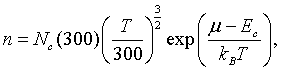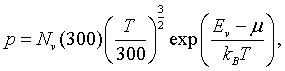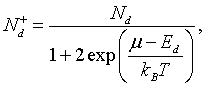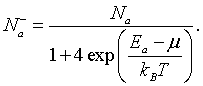## Carrier concentration vs. Fermi energy

For a doped semiconductor, the density of electrons in the conduction band is,the density of holes in the valence band is,the density of ionized donors is,and the density of ionized acceptors is,The factor of 4 is valid in the formula for the acceptors if the semiconductor has a light hole and a heavy hole band as Si and Ge do.

The four quantities n, p, Nd, and Na can only be determined if the chemical potential, μ, is known. Typically, μ must first be determined from the charge neutrality condition,

n + Na- = p + Nd+.

The chemical potential can be found by solving the charge neutrality condition numerically. One way to do this is to program the formulas for n, p, Nd+, and Na- in a spreadsheet. Then choose a temperature and calculate n, p, Nd+, Na- for every value of the chemical potential between Ev and Ec. For one of these μ values, the charge neutrality condition will be satisfied.

When n + Na- and , p + Nd+ are ploted as a function of μ, the chemical potential is where the two lines cross.

 log [1/cm³] μ [eV]
 Nc(300 K) = 1/cm³ Nv(300 K) = 1/cm³ Nd = 1/cm³ Na = 1/cm³ Ed = eV Ea = eV Eg = eV T = K
```The plot is generated with the following code:

for (i=0; i<500; i++) {
Ef = i*Eg/500;
n=Nc*pow(T/300,1.5)*exp(1.6022E-19*(Ef-Eg)/(1.38E-23*T));
p=Nv*pow(T/300,1.5)*exp(1.6022E-19*(-Ef)/(1.38E-23*T));
Namin = Na/(1+4*exp(1.6022E-19*(Ea-Ef)/(1.38E-23*T)));
Ndplus = Nd/(1+2*exp(1.6022E-19*(Ef-Ed)/(1.38E-23*T)));
}

```